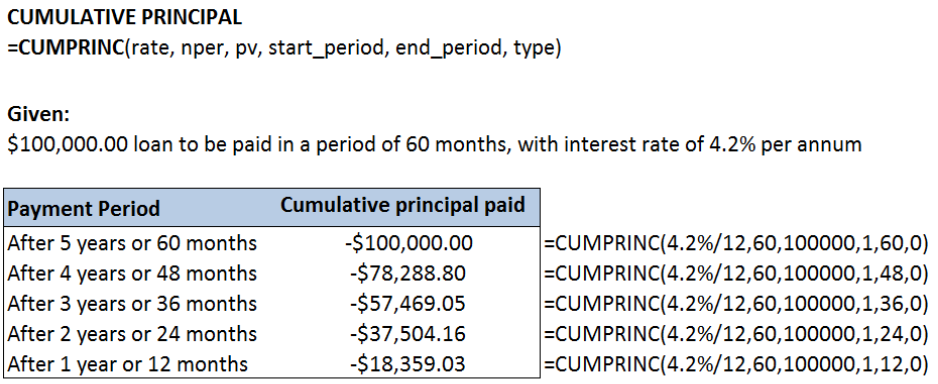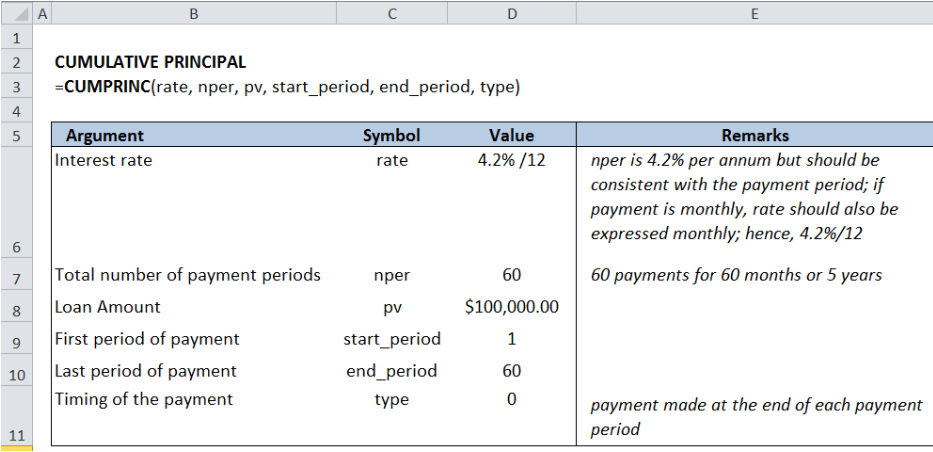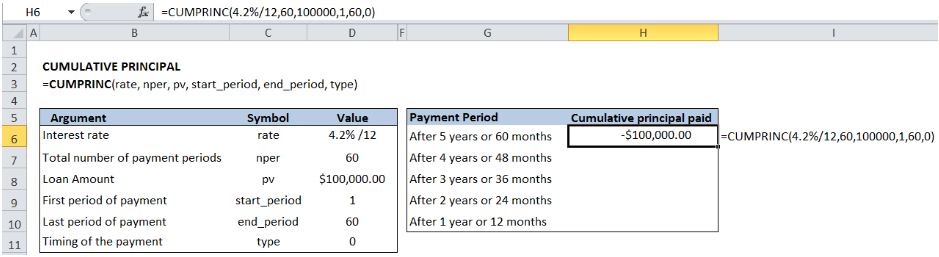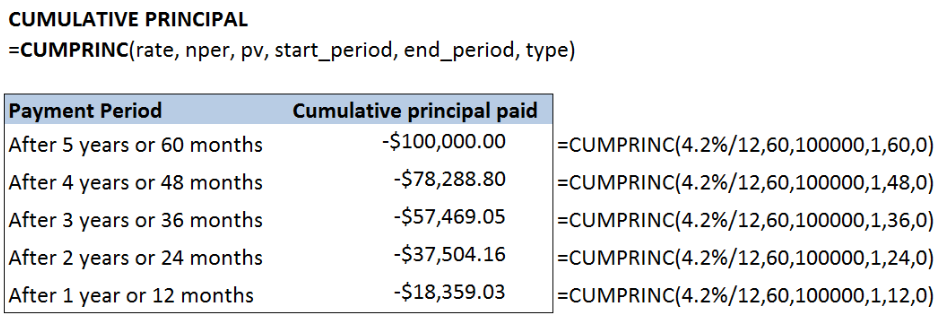Get instant live expert help with Excel or Google Sheets“My Excelchat expert helped me in less than 20 minutes, saving me what would have been 5 hours of work!”

#### Post your problem and you'll get expert help in seconds

Your message must be at least 40 characters
Our professional experts are available now. Your privacy is guaranteed.

# Excel CUMPRINC Function

When paying off a loan, we often wonder how much of the principal amount have we paid off already.  There is a financial function in Excel that calculates the cumulative principal paid over a period of time, the CUMPRINC function. This step by step tutorial will assist all levels of Excel users in calculating the remaining principal balance on a loan.Figure 1. Output: CUMPRINC function in Excel

CUMPRINC function returns the cumulative principal paid on a loan over a specified period of payments

## Syntax

`=CUMPRINC(rate, nper, pv, start_period, end_period, type)`

Where

• Rate: interest rate;
• Nper: total number of payment periods
• Pv: loan amount
• Start_period: First period of payment in the calculation
• End_period: Last period of payment in the calculation
• Type: timing of the payment
• 0 (zero) – payment at the end of the period
• 1 – payment at the start of the period

## Setting up the Data

Suppose we have the following data for our calculation:

\$100,000.00 loan to be paid in a period of 60 months, with interest rate of 4.2% per annum

Below table shows the values for each argument of the CUMPRINC function, according to the given data above.Figure 2.  Sample data for CUMPRINC function in Excel

## Using CUMPRINC Function in Excel

We want to calculate the cumulative principal paid on a loan over the whole period of the loan, which is 60 months.  It is expected that the full amount should be paid off after the whole payment period.

In cell H6, enter the formula:

`=CUMPRINC(4.2%/12,60,100000,1,60,0)`Figure 3.  Entering the formula for CUMPRINC function in Excel

This formula results to -\$100,000.00.  This confirms that after the whole payment period, the full principal amount has been paid off.

Note that the result of the CUMPRINC function is a negative value, since the principal amount paid is an outgoing cash flow.

## Important Notes

• The  loan amount should be a positive value
• The interest rate should be consistent with the payment period; in this case, since the payment is monthly, the interest rate should also be monthly (e.g. 4.2% /12)

The table below shows the cumulative principal paid on a loan for every year of payment.Figure 4.  Final result: Calculate cumulative principal using CUMPRINC function in Excel

Most of the time, the problem you will need to solve will be more complex than a simple application of a formula or function. If you want to save hours of research and frustration, try our live Excelchat service! Our Excel Experts are available 24/7 to answer any Excel question you may have. We guarantee a connection within 30 seconds and a customized solution within 20 minutes.